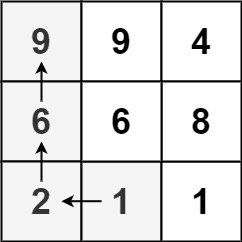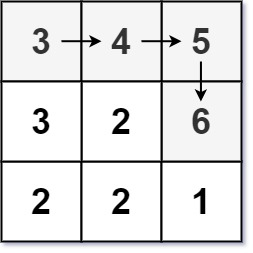# 329. Longest Increasing Path in a Matrix | LeetCode Solution

## 329. Longest Increasing Path in a Matrix | LeetCode Solution

Given an `m x n` integers `matrix`, return the length of the longest increasing path in `matrix`.

From each cell, you can either move in four directions: left, right, up, or down. You may not move diagonally or move outside the boundary (i.e., wrap-around is not allowed).

Example 1:```Input: matrix = [[9,9,4],[6,6,8],[2,1,1]]
Output: 4
Explanation: The longest increasing path is `[1, 2, 6, 9]`.
```

Example 2:```Input: matrix = [[3,4,5],[3,2,6],[2,2,1]]
Output: 4
Explanation: The longest increasing path is `[3, 4, 5, 6]`. Moving diagonally is not allowed.
```

Example 3:

```Input: matrix = []
Output: 1
```

Constraints:

• `m == matrix.length`
• `n == matrix[i].length`
• `1 <= m, n <= 200`
• `0 <= matrix[i][j] <= 231 - 1`

class Solution {
public:
int ans=0;
int helper(vector<vector<int>>&v, vector<vector<int>>&dp, int i,int j){
if(i<0 || j<0 || i>=v.size() || j>=v.size())return 0;

if(dp[i][j]!=-1)return dp[i][j];
int left=0,right=0,top=0,down=0;
if(i>0 && v[i-1][j]>v[i][j])left+=helper(v,dp,i-1,j)+1;
if(j>0 && v[i][j-1]>v[i][j])top+=helper(v,dp,i,j-1)+1;
if(i<v.size()-1 && v[i+1][j]>v[i][j])down+=helper(v,dp,i+1,j)+1;
if(j<v.size()-1 && v[i][j+1]>v[i][j])right+=helper(v,dp,i,j+1)+1;

top=max(top,down);
left=max(left,right);
dp[i][j]=max(top,left);
ans=max(ans,dp[i][j]);
return dp[i][j];
}

int longestIncreasingPath(vector<vector<int>>& matrix) {
vector<vector<int>>dp(matrix.size(),vector<int>(matrix.size(),-1));
for(int i=0; i<matrix.size(); i++){
for(int j=0; j<matrix.size(); j++)
helper(matrix,dp,i,j);
}
return ans+1;
}
};